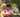### Cyclic Rotation

January 16, 2020

CyclicRotation

Write a function:
function solution(A, K);
that, given an array A consisting of N integers and an integer K, returns the array A rotated K times.

For example, given
A = [3, 8, 9, 7, 6] K = 3
the function should return [9, 7, 6, 3, 8]. Three rotations were made:
[3, 8, 9, 7, 6] -> [6, 3, 8, 9, 7]
[6, 3, 8, 9, 7] -> [7, 6, 3, 8, 9]
[7, 6, 3, 8, 9] -> [9, 7, 6, 3, 8]

For another example, given
A = [0, 0, 0]
K = 1
the function should return [0, 0, 0]

Given
A = [1, 2, 3, 4]
K = 4
the function should return [1, 2, 3, 4]

Assume that:
• N and K are integers within the range [0..100];
• each element of array A is an integer within the range [−1,000..1,000].

This problem can be solved by using array's slice function. Be advised that K could be bigger than array's length.

``````function solution(A, K) {
// write your code in JavaScript (Node.js 8.9.4)
const len = A.length
let p = 0

if(K == len) return A           // rotation steps = array length
if(K > len) p = len - (K % len) // rotation steps > array length
else p = len - K

const array1 = A.slice(p)
const array2 = A.slice(0, p)

return array1.concat( array2 )
}

console.log("solution([3, 8, 9, 7, 6], 3): ", solution([3, 8, 9, 7, 6], 3))``````Posted by Tai Lu ( 呂台生 ) who's building useful things.You should follow him on Twitter